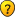awing_94
Posts: 11
Joined: Mon Jun 25, 2007 3:56 am

### urgent...pls

why is the work outputlies when a exerted at an angle than when the force is exerted parallel to the direction of motion:?:pls...i really need help..imediately!louise_awing

tdaly
Former Expert
Posts: 1415
Joined: Sat Nov 08, 2003 11:27 pm
Occupation: Planetary Scientist
awing_94,

First, let me just give you a tip that will make your life in these forums a whole lot easier. You need to understand that most experts only check these forums once a week. A few of us check more often than that, but dn't expect an immediate response. The average response time is between 24 and 48 hours.

So, to your question. To be honest, I'm not sure I understand what you are asking. It seems to me like you are asking about work. Is this correct? Do you mean work in the formal sense (a force exerted through a distance) or are you using work to mean something else? Are you discussing translational motion or rotatation? Without more information, I really can't answer your question.

Here's some general information about work, as the term is used in physics. Work is done by exerting a force through a distance. Thus, work (W) is the product of force (F) and distance (d). So, we can say that W = Fd. However, forces are vector quantities (are do you know the difference between vector and scalar quantities?) and so work itself is a vector quantity. This means that we must not only consider the magnitude of the force (how "hard" we are pushing or pulling) but also its direction (left, right, East, West, etc.) So, as the angle of the exerted force relative to the direction of translation varies, differing amounts of work are done.

All the best,
Terik

ThePinkRocker
Posts: 3
Joined: Thu Sep 27, 2007 9:55 pm
I think your question is ___"Why is the work output less when the force is exerted at an angle than when the force is exerted parallel to the direction of motion?"
Is that your question? If it is, then the answer is quite simple.

We know that work is a vector quantity (i.e. it posseses both magnitude and direction). So even if the direction of of the force is change, keeping the magnitude constant, the resulting work done is affected. This can be shown by the following calculation ____

When th force is applied in the direction of motion, and the object is in a steady state of motion, then he wok is given by

W = FD [where, F=Force applied; D=Displacement of the body]
Note that, here, the force is acting parallel to the direction of motion. And since F and D are both vectors, their Dot product gives us the above result.

That is___

W = F.D
= FDcos@
[where, @ = The angle between the direction of the force applied and the horizontal]

But here, since the force is parallel to the horizontal, therefore,
@ = zero degrees.

Which gives us the result W = FD [Since cos(0) = 1]

But, if the force is exerted at an angle, say 60 degrees, then the value of work reduces, as shown below___
(Lei the new work done be W")
W" = FDcos(60)
= FD(0.5)
Which means that in the above example, the work is reduced to half.

Thus, the work output is less when a force is applied at an angle than when it is exerted parallel to the direction of the motion of the body.

Cheers & Best of Luck.